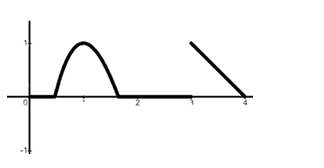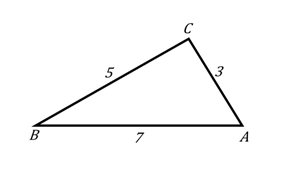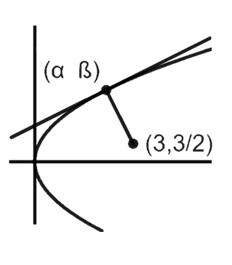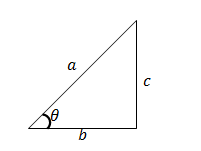Checkout JEE MAINS 2022 Question Paper Analysis : Checkout JEE MAINS 2022 Question Paper Analysis :

# JEE Main 2021 July 20 Shift 2 Maths Question Paper

JEE Main 2021 July 20 shift 2 Maths question paper is a perfect resource that will help candidates in clearly understanding the overall JEE Main session 3 question paper blueprint. Candidates can view or download the Maths question paper along with the solutions given here to figure out the important concepts and their weightage in the entrance examination.
Practising solving the different types of questions will enable the JEE aspirants to predict the type of questions that can appear in the exam. It will further motivate them to face the exam with adept problem-solving skills and higher confidence. Solving the JEE Main 2021 July 20 shift 2 Maths question paper will also help students gain knowledge about the time required for each question, how to find the right approach and select the right answer only.

### July 20 Shift 2 - Maths Question Paper and Solutions

Question 1:-π/2π/2([x] - [sin⁡x ])dx = (Where [.] represents G.I.F)

1. a. -2
2. b. 1
3. c. 0
4. d. -1

Solution:

I = ∫-π/2π/2([x] - [sin⁡x ])dx

Using the property ∫-aa f(x)dx = ∫0a f(x)dx + ∫0a f(-x)dx

I = ∫0π/2 ([x] + [-x] )dx - ∫0π/2([sin ⁡x] + [-sin ⁡x])dx

= 0

Hence option c is the answer.

Question 2: If

$$\lim_{x\to 0}\frac{\alpha x(1+x+\frac{x^{2}}{2!}+\frac{x^{3}}{3!}+...)-\beta (x-\frac{x^{2}}{2}+\frac{x^{3}}{3}+...)+\gamma x^{2}(1-x+\frac{x^{2}}{2!}-\frac{x^{3}}{3!}+...)}{x^{3}}=10$$
, find the value of α + β + γ

Solution:

$$\lim_{x\to 0}\frac{\alpha x(1+x+\frac{x^{2}}{2!}+\frac{x^{3}}{3!}+...)-\beta (x-\frac{x^{2}}{2}+\frac{x^{3}}{3}+...)+\gamma x^{2}(1-x+\frac{x^{2}}{2!}-\frac{x^{3}}{3!}+...)}{x^{3}}=10$$

⇒ α - β = 0

⇒ α = β

⇒ α + β/2 + γ = 0

⇒ γ = -3β/2

⇒ α/2 - β/3 - γ = 10

⇒ β = 6, α = 6, γ = -9

So, the value of α + β + γ = 3

Question 3: The value of x satisfying the equation log(x+1)⁡(2x2+7x+5) + log(2x+5)⁡(x+1)2 = 4 is:

1. a. -2
2. b. 2
3. c. -4
4. d. 4

Solution:

log(x+1) ⁡(2x+5)(x+1) + log(2x+5) (x+1)2 = 4

1+ log(x+1) (2x+5)⁡ + 2log(2x+5) (x+1) = 4

Put log(x+1) (2x+5) = t

∴1+ t + 2/t = 4

⇒ t2 + t + 2 = 4t

⇒ t2 - 3t + 2 = 0

t = 1, t = 2

For t = 1

2x+5 = x + 1

⇒ x = -4 (Rejected)

For t = 2

2x+5 = (x + 1)2

x = 2, x = -2 (Rejected)

Hence option b is the answer.

Question 4: Mean of 6 observations is 10 and their variance is 20/3 . If observations are 15, 11, 10, 7, a, b then |a-b| is equal to:

1. a. 2
2. b. 1
3. c. 3
4. d. 4

Solution:

Mean = 10

(7+10+11+15+a+b)/6 = 10

a + b = 17

Variance = 20/3

(49+100+121+225+a2+b2)/6 - 100 = 20/3

a2+b2 = 145

(a+b)2 = 289

ab = 72

(a-b)2 = (a+b)2 - 4ab

(a-b)2 = 289 - 288 = 1

|a-b| = 1

Hence option b is the answer.

Question 5: If f(x) = x+1, then find

$$\lim_{n\to \infty }\frac{1}{n}\left [ f(0)+f(\frac{5}{n})+ f(\frac{10}{n})+...+f(\frac{5(n-1)}{n})\right ]$$

1. a. 7/2
2. b. 3/2
3. c. 5/2
4. d. 1/2

Solution:

$$\lim_{n\to \infty }\frac{1}{n}\sum_{r=0}^{n-1}f(\frac{5r}{n})$$

= ∫01 f(5x)

= ∫01(5x+1) dx

= ((5x2/2) + x)01

= (5/2) + 1

= 7/2

Hence option a is the answer.

Question 6: Sum of 21 terms of series

$$log_{9^{\frac{1}{2}}}x + log_{9^{\frac{1}{3}}}x + log_{9^{\frac{1}{4}}}x..........$$
is 252, then the value of x is:

1. a. 7
2. b. 243
3. c. 9
4. d. 81

Solution:

2 log9 x + 3 log9 x + 4 log9 x………….21 terms

= (2 + 3 + 4 + 5…+ 22) log9x

= (21/2)(2 + 22) log9x

= 21×12 log9x

= 252 log9x

Given sum = 252

⇒ log9 x = 1

⇒ x = 9

Hence option c is the answer.

Question 7: If Re [(1+cos θ + 2i sin⁡ θ)] = 4, then the value of θ is

1. a. π/2
2. b. π/3
3. c. -π/2
4. d. π

Solution:

[1/(1 + cos2θ + 2i sin θ)] ×(1+cos θ - 2i sin θ)/(1 + cos θ - 2i sin θ)

= (1 + cos θ - 2i sin θ)/((1 + cos θ)2+ 4 sin2θ)

⇒ (1 + cos θ)/(1 + cos2θ + 2 cos θ + 4 sin2θ) = 4

⇒ (1 + cos θ)/(1 + cos2θ + 2cosθ + 4 - 4 cos2θ) = 4

⇒ (1 + cos θ)/(5 + 2 cos θ - 3 cos2 θ) = 4

⇒ 1 + cos θ = 20 + 8 cos θ - 12 cos2 θ

12cos2θ - 7cos θ -19 = 0

⇒ 12cos2θ -19cos θ + 12cos θ - 19 = 0

⇒ cos θ(12cos θ -19) + 1(12cos θ -19) = 0

⇒ cos θ = -1 or cosθ = 19/12 (rejected)

⇒ θ = π

Hence option d is the answer.

Question 8: If x = ay-1 = z-2, and x = 3y-2 = bz-2 lie in same plane, then the value of a, b is

1. a. a = 2,b = 3
2. b. a = 1, b = 1
3. c. b = 1, a ∈ R-{0}
4. d. a = 3,b = 2

Solution:

(x/1) = (y-1/a)/(1/a) = (z-2)/1

x = (y-⅔)/(⅓) = (z-2/b)/(1/b)

$$(\vec{a_{1}}-\vec{a_{2}}).(\vec{b_{1}}-\vec{b_{2}})=0$$

$$\begin{vmatrix} 0 &\frac{1}{a}-\frac{2}{3} &2-\frac{2}{b} \\ 1 & \frac{1}{a} &1 \\ 1& \frac{1}{3}& \frac{1}{b} \end{vmatrix}=0$$

⇒ (1/ab) - (1/a) = 0

⇒ b = 1, a∈ R-{0}

Hence option c is the answer.

Question 9: If

$$P(\bar{A}\cap B)+P(A\cap \bar{B})= 1-k$$
,

$$P(\bar{A}\cap C)+P(A\cap \bar{C})= 1-2k$$
,

$$P(\bar{B}\cap C)+P( \bar{C}\cap B)= 1-k$$

P(A∩B∩C) = k2, k ∈(0, 1). Then the value of P (at least one of A, B, C) is

1. a. >1/2
2. b. [⅛, ¼]
3. c. < 1/4
4. d. 1/4

Solution:

P(A) + P(B) - 2P(A∩B) = 1 - k

P(A) + P(C) - 2P(A∩C) = 1 - 2k

P(B) + P(C) - 2P(B∩C) = 1 - k

P(A⋃B⋃C) = P(A) + P(B) + P(C) - P(A∩B) - P(B∩C) - P(A∩C) + P(A∩B∩C)

= (3 - 4k)/2) + k2

= (2k2 - 4k + 3)/2

Since the value of 2k2 - 4k + 3 is greater than 1

P(A⋃B⋃C) = 1/2

Hence option a is the answer.

Question 10: If f(x) = (5x+3)/(6x+a) and f(f(x) = x then the value of a is:

1. a. -5
2. b. 5
3. c. 6
4. d. -6

Solution:

f(f(x) = (5f(x)+3)/(6f(x)+a) = x

⇒ 5f(x)+3 = 6xf(x)+ax

[(25x+15)/(6x+a)] + 3 = 6x((5x+3)/(6x+a)) + ax

⇒ 25x+15+18x+3a = 30x2+18x+6ax2+a2x

⇒(30+6a)x2 + (a2-25)x - (3a+15)=0

⇒6(a+5)x2 + (a2-25)x - (3a+15) = 0,

a + 5 = 0

a = -5

Hence option a is the answer.

Question 11: If

$$g(t)=\begin{Bmatrix} max(t^{3}-6t^{2}+9t-3,0), & t \; belongs\: to\: [0,3] \\ 4-t & t \; belongs\: to\:(3,4) \end{Bmatrix}$$
then the number of points at which g(t) is non differentiable is:

1. a. 1
2. b. 3
3. c. 2
4. d. 4

Solution:y = t3- 6t2 + 9t - 3

y’ = 3t2-12t + 9

= 3(t2- 4t + 3)

So total 3 points.

Hence option b is the answer.

Question 12: Given below are three conditions:

A: If 2+4 = 7, then 3+4 = 8

B: if 3+5 = 8, then earth is flat

C: if A and B are true, then 5+4 = 11

Choose the correct option.

Solution:

Truth table p → q

 p q p→ q T T T T F F F T T F F T

A is true, B is false, C is true

Hence option d is the answer.

Question 13: If A =

$$\begin{bmatrix} 1 & 0\\ 0& 1 \end{bmatrix}$$
1
$$B\sum_{r=1}^{2021}A^{r}$$
then value of |B| is

1. a. 2021
2. b. 20212
3. c. -2021
4. d. 0

Solution:

A = I, B= I+I+I+....,2021 times

$$B=2021\begin{bmatrix} 1 & 0\\ 0& 1 \end{bmatrix}$$

|B| = 20212

Hence option b is the answer.

Question 14: If element of matrix A = [aij](3×3) where

$$A = \left\{\begin{matrix} (-1)^{j-i} &i<j \\ 2& i=j\\ (-1)^{i+j}& i>j \end{matrix}\right.$$
, then the value of |3 adj(2A-1)| is:

1. a. 72
2. b. 36
3. c. 108
4. d. 48

Solution:

A =

$$\begin{bmatrix} 2 & -1 & 1\\ -1& 2& -1\\ 1& -1& 2 \end{bmatrix}$$

|A| = 2(4-1)+1(-2+1)+1(1-2)

= 2(3)+1(-1)+1(-1)

= 4

= 33×|2A-1|2

= 33×26×|A−1|2

= 33×26×1/|A|2

= 108

Hence option c is the answer.

Question 15: In a ∆ABC, if

$$\left | \overrightarrow{AB} \right |=7$$
,
$$\left | \overrightarrow{BC} \right |=5$$
, and
$$\left | \overrightarrow{CA} \right |=3$$
. If the projection of
$$\overrightarrow{BC}$$
on
$$\overrightarrow{CA}$$
is n/2, then the value of n is

Solution:

$$\left | \overrightarrow{AB} \right |=7$$

$$\left | \overrightarrow{BC} \right |=5$$

$$\left | \overrightarrow{CA} \right |=3$$Projection of

$$\overrightarrow{BC}$$
on
$$\overrightarrow{CA}$$
is =
$$\left | \overrightarrow{BC} \right |$$
cos ∠BCA

5|(32+52-72)/(2.3.5)| = 5|-15/30|

= 5/2

Question 16: The value of tan (2 tan-1 (3/5) + sin-1(5/13)) is:

1. a. 220/21
2. b. 110/21
3. c. 55/21
4. d. 20/11

Solution:

tan (tan-1 (6/5)/(1-(9/5)) + tan-1 (5/12))

= tan (tan-1 (15/8) + tan-1 (5/12))

= ((15/8) + (5/12))/(1 - (15/8)(5/12)

= 220/21

Hence option a is the answer.

Question 17: If (α, β) is the point on y2 = 6x, that is closest to (3, 3/2) then find 2(α+β)

1. a. 6
2. b. 9
3. c. 7
4. d. 5

Solution:Equation of normal at (α, β) is

y-β = (-β/2a)(x-α)

⇒ 3y-3β = -β(x-α)

For shortest distance normal will pass through (3, 3/2)

So (9/2) - 3β = -3β-βα

αβ = 9/2⋯(1)

Now from y2 = 6x

β2 = 6α⋯(2)

From (1) and (2) we get α = 3/2, β = 3

2(α+β) = 2(3/2 + 3)

= 2(9/2)

= 9

Hence option b is the answer.

Question 18: Two circles pass through (-1, 4) and their centres lie on x2+y2+2x+4y = 4. If r1 and r2 are maximum and minimum radii and r1/r2 = a+b√2, then the value of a+b is

Solution:

Given circle

(x+1)2+(y+2)2 = 32

Any point on the circle is (3 cos θ - 1,3 sin θ - 2)

Radius of the circles which passes through (-1, 4)

r = √((3 cos θ - 1 + 1)2 + (3 sin θ - 2 - 4)2)

=√(9 cos2 θ + 9 sin2 θ + 36 - 36 sin θ)

= √(45 - 36 sin θ)

rmax = 9 = r1 and rmin = 3 = r2

⇒ r1/r2 = 9/3 = 3

⇒ 3 + 0√2

= a+b

= 3

Question 19: If ∆ABC is a right-angled triangle with sides a,b and c and smallest angle θ. If 1/a, 1/b and 1/c are also the sides of the right-angle triangle then find sin θ.

Solution:

Let a>b>c

sin θ = c/a1/a < 1/b < 1/c

1/c2 = 1/a2 + 1/b2

1 = (c2/a2) + (c2/b2)

1 = (c2/a2) + (c2/(a2 - c2)) (As b2 + c2 = a2)

1 = sin2θ + 1/((a2/c2)-1)

= (sin2 θ) + (1/(cosec2θ-1))

(1-sin2 θ + 1)/(cosec2 θ -1) = 1

⇒ sin2 θ + cosec2 θ = 3

Put sin2 θ = t

⇒ t4 - 3t2 + 1 = 0

⇒ t2 = (3±√5)/2

So sin θ = √((3-√5)/2)

Hence option a is the answer.

### JEE Main 2021 Maths Paper July 20 Shift 2